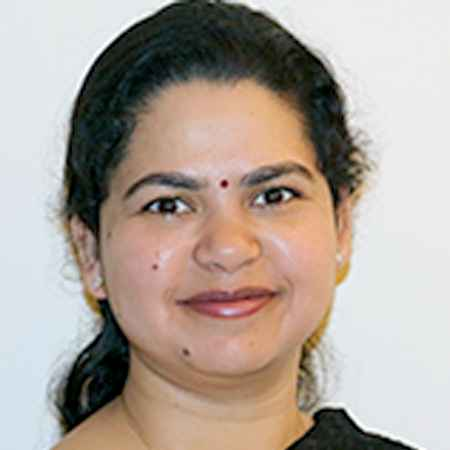X
Improving classifier fusion via Pool Adjacent Violators normalization
G. Goswami, N. Ratha,
Published in Institute of Electrical and Electronics Engineers Inc.
2016
Volume: 0

Pages: 1011 - 1016
Abstract
Classifier fusion is a well-studied problem in which decisions from multiple classifiers are combined at the score, rank, or decision level to obtain better results than a single classifier. Subsequently, various techniques for combining classifiers at each of these levels have been proposed in the literature. Many popular methods entail scaling and normalizing the scores obtained by each classifier to a common numerical range before combining the normalized scores using the sum rule or another classifier. In this research, we explore an alternative method to combine classifiers at the score level. The Pool Adjacent Violators (PAV) algorithm has traditionally been utilized to convert classifier match scores to confidence values that model posterior probabilities for test data. The PAV algorithm and other score normalization techniques have studied the same problem without being aware of each other. In this first ever study to combine the two, we propose the PAV algorithm for classifier fusion on publicly available NIST multi-modal biometrics score dataset. We observe that it provides several advantages over existing techniques and find that the interpretation learned by the PAV algorithm is more robust than the scaling learned by other popular normalization algorithms such as min-max. Moreover, the PAV algorithm enables the combined score to be interpreted as confidence and is able to further improve the results obtained by other approaches. We also observe that utilizing traditional normalization techniques first for individual classifiers and then normalizing the fused score using PAV offers a performance boost compared to only using the PAV algorithm. © 2016 IEEE.
••